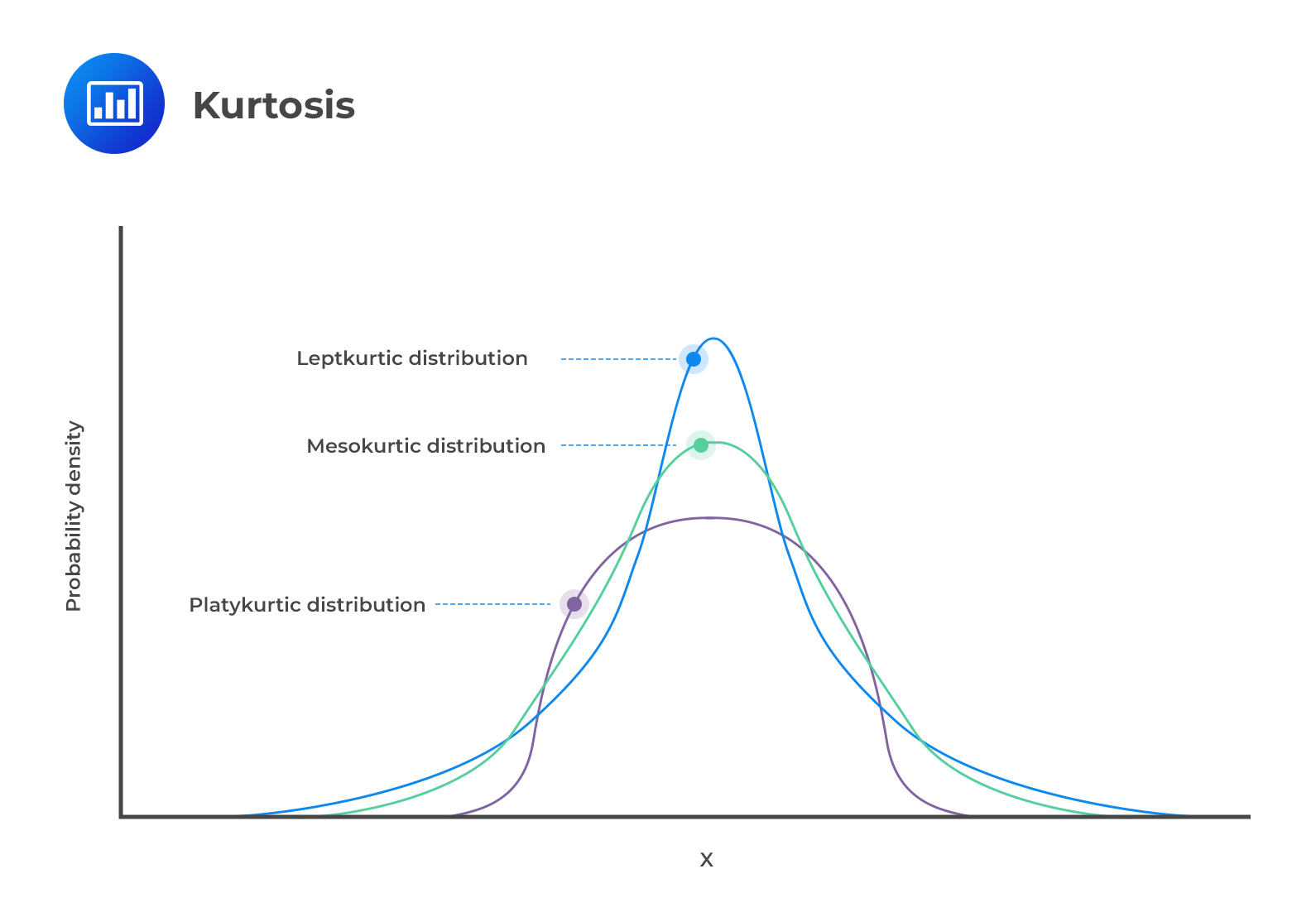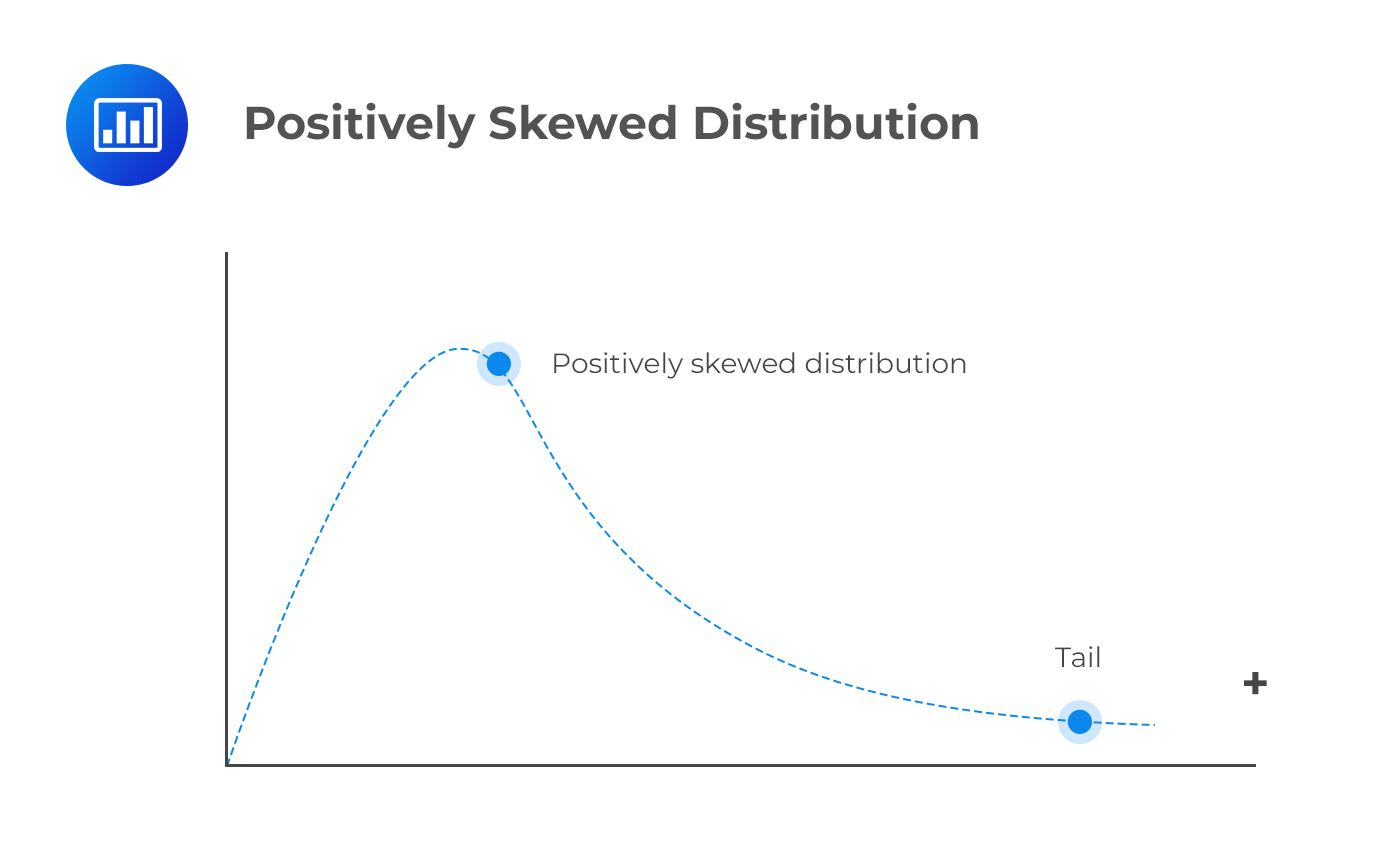# Kurtosis

Kurtosis refers to the measurement of the degree to which a given distribution is more or less ‘peaked’ relative to the normal distribution. The concept of kurtosis is very useful in decision-making.  In this regard, we have 3 categories of distributions:

• Leptokurtic;
• Mesokurtic; or
• Platykurtic.## Leptokurtic

A leptokurtic distribution is more peaked than the normal distribution. The higher peak results from the clustering of data points along the x-axis. The tails are also fatter than those of a normal distribution. The coefficient of kurtosis is usually more than 3.

The term “lepto” means thin or skinny. When analyzing historical returns, a leptokurtic distribution means that small changes are less frequent since historical values are clustered around the mean. However, there are also large fluctuations represented by the fat tails.

## Platykurtic

A platykurtic distribution has extremely dispersed points along the x-axis, resulting in a lower peak when compared to a normal distribution. “Platy” means broad. Hence, the prefix fits the distribution’s shape, which is wide and flat. The points are less clustered around the mean compared to a leptokurtic distribution. The coefficient of kurtosis is usually less than 3.

Returns that follow this type of distribution have fewer major fluctuations compared to leptokurtic returns. However, you should note that fluctuations represent the riskiness of an asset. More fluctuations represent more risk and vice versa. Therefore, platykurtic returns are less risky than leptokurtic returns.

## Mesokurtic

Lastly, mesokurtic distributions have a curve that is similar to that of a normal distribution. In other words, the distribution is largely normal.

## Measures of Sample Skewness and Kurtosis

Exam tip: The learning outcome statement reads, explain measures of sample skewness and kurtosis.” However, the calculations will have you better understand those concepts.

### Sample Skewness

$${ S }_{ k }=\frac { 1 }{ n } \frac { \sum _{ i=1 }^{ n }{ { \left( { X }_{ i }-\bar { X } \right) }^{ 3 } } }{ { S }^{ 3 } }$$

Where:

$$\bar { X }$$ = Sample mean;

$$S$$ = Sample standard deviation; and

$$n$$ = Number of observations.

Note: The formula is very similar to the formula for standard deviation, but raised to the 3rd power.

Interpretation: A positive value indicates positive skewness. A ‘zero’ value indicates that the data is not skewed. Lastly, a negative value indicates negative skewness or rather a negatively skewed distribution.

### Sample Kurtosis

Sample kurtosis is always measured relative to the kurtosis of a normal distribution, which is 3. Therefore, we are always interested in the “excess“ kurtosis, i.e.,

$$\text{ Excess kurtosis = Sample kurtosis – 3 }$$, where:

$${ S }_{ kr }=\frac { 1 }{ n } \frac { \sum _{ i=1 }^{ n }{ { \left( { X }_{ i }-\bar { X } \right) }^{ 4 } } }{ { S }^{ 4 } }$$

Interpretation: Positive excess kurtosis indicates a leptokurtic distribution. A zero value indicates a mesokurtic distribution. Lastly, a negative excess kurtosis represents a platykurtic distribution.

#### Example: Calculating Skewness

Suppose we have the following observations:

{ 12   13   54   56   25 }

What is the skewness of the data?

Solution

First, we must determine the sample mean and the sample standard deviation:

$$X =\cfrac {(12 + 13 +…+25)}{5} =\cfrac {160}{5} = 32$$

$$S^2= \cfrac{{(12 – 32)^2 + …+ (25 – 32)^2}}{4} = 467.5$$

Therefore,

$$S = 467.5^{\frac{1}{2}} = 21.62$$

Now we can work out the skewness:

$${ S }_{ k }=\frac { 1 }{ n } \frac { \sum _{ i=1 }^{ n }{ { \left( { X }_{ i }-\bar { X } \right) }^{ 3 } } }{ { S }^{ 3 } } =\frac { 1 }{ 5 } \frac { { -20 }^{ 3 }+{ (-19 }^{ 3 })+{ 22 }^{ 3 }+{ 24 }^{ 3 }+{ (-7 }^{ 3 }) }{ { 21.62 }^{ 3 } } =0.1835$$

Skewness is positive. Hence, the data has a positively skewed distribution.

#### Example: Calculating Kurtosis

Using the data from the example above { 12   13   54   56   25 }, determine the type of kurtosis present.

Solution

$$X =\cfrac {(12 + 13 +…+25)}{5} =\cfrac {160}{5} = 32$$

$$S^2=\cfrac {{(12 – 32)^2 + …+ (25 – 32)^2}}{4} = 467.5$$

Therefore,

$$S = 467.5^{\frac{1}{2}} = 21.62$$

$${ S }_{ kr }=\frac { 1 }{ n } \frac { \sum _{ i=1 }^{ n }{ { \left( { X }_{ i }-\bar { X } \right) }^{ 4 } } }{ { S }^{ 4 } } =\frac { 1 }{ 5 } \frac { { -20 }^{ 4 }+{ (-19 }^{ 4 })+{ 22 }^{ 4 }+{ 24 }^{ 4 }+{ (-7 }^{ 4 }) }{ { 21.62 }^{ 4 } } =0.7861$$

Next, we subtract 3 from the sample kurtosis and get the excess kurtosis:

$$\text {Excess kurtosis} = 0.7861 – 3 = -2.2139$$

Since the excess kurtosis is negative, we have a platykurtic distribution.

## Question 1

The skewness of the normal distribution is most likely:

1. zero.
2. positive.
3. negative.

Solution

Since the normal curve is symmetric about its mean, its skewness is zero.

B is incorrect because a positively skewed distribution has positive skewness.

C is incorrect because a negatively skewed distribution has negative skewness.

## Question 2

A frequency distribution in which there are too few scores at the extremes of the distribution is most likely called:

1. platykurtic.
2. leptokurtic.
3. mesokurtic.

Solution

A platykurtic distribution has “thin” tails and is flatter compared to a normal distribution. It implies that there are fewer scores at the extremes of the distribution, which aligns with the question’s description.### Question 3

When most of the data are concentrated on the left of the distribution, it is most likely called:

1. symmetric distribution.
2. positively skewed distribution.
3. negatively skewed distribution.

Solution

A distribution is said to be skewed to the right, or positively skewed, when most of the data are concentrated on the left of the distribution. A distribution is said to be skewed to the left, or negatively skewed, if most of the data are concentrated on the right of the distribution. The left tail clearly extends farther from the distribution’s center than the right tail.A is incorrect. A symmetric distribution is one in which the left and right sides mirror each other.

C is incorrect. A distribution is said to be skewed to the left, or negatively skewed, if most of the data are concentrated on the right of the distribution. The left tail extends farther away from the mean than the right tail.

Shop CFA® Exam Prep

Offered by AnalystPrepLevel I
Level II
Level III
All Three Levels
Featured Shop FRM® Exam PrepFRM Part I
FRM Part II
FRM Part I & Part II
Learn with Us

Subscribe to our newsletter and keep up with the latest and greatest tips for success
Shop Actuarial Exams PrepExam P (Probability)
Exam FM (Financial Mathematics)
Exams P & FM
Shop GMAT® Exam PrepComplete CourseSergio Torrico
2021-07-23
Excelente para el FRM 2 Escribo esta revisión en español para los hispanohablantes, soy de Bolivia, y utilicé AnalystPrep para dudas y consultas sobre mi preparación para el FRM nivel 2 (lo tomé una sola vez y aprobé muy bien), siempre tuve un soporte claro, directo y rápido, el material sale rápido cuando hay cambios en el temario de GARP, y los ejercicios y exámenes son muy útiles para practicar.diana
2021-07-17
So helpful. I have been using the videos to prepare for the CFA Level II exam. The videos signpost the reading contents, explain the concepts and provide additional context for specific concepts. The fun light-hearted analogies are also a welcome break to some very dry content. I usually watch the videos before going into more in-depth reading and they are a good way to avoid being overwhelmed by the sheer volume of content when you look at the readings.Kriti Dhawan
2021-07-16
A great curriculum provider. James sir explains the concept so well that rather than memorising it, you tend to intuitively understand and absorb them. Thank you ! Grateful I saw this at the right time for my CFA prep.nikhil kumar
2021-06-28
Very well explained and gives a great insight about topics in a very short time. Glad to have found Professor Forjan's lectures.Marwan
2021-06-22
Great support throughout the course by the team, did not feel neglectedBenjamin anonymous
2021-05-10
I loved using AnalystPrep for FRM. QBank is huge, videos are great. Would recommend to a friendDaniel Glyn
2021-03-24
I have finished my FRM1 thanks to AnalystPrep. And now using AnalystPrep for my FRM2 preparation. Professor Forjan is brilliant. He gives such good explanations and analogies. And more than anything makes learning fun. A big thank you to Analystprep and Professor Forjan. 5 stars all the way!michael walshe
2021-03-18
Professor James' videos are excellent for understanding the underlying theories behind financial engineering / financial analysis. The AnalystPrep videos were better than any of the others that I searched through on YouTube for providing a clear explanation of some concepts, such as Portfolio theory, CAPM, and Arbitrage Pricing theory. Watching these cleared up many of the unclarities I had in my head. Highly recommended.